# Automatic Recognition of Rock Images Based on Convolutional Neural Network and Discrete Cosine Transform

Automatic Recognition of Rock Images Based on Convolutional Neural Network and Discrete Cosine Transform
Shandong Agriculture and Engineering University, Ji’nan 250100, China

Corresponding Author Email:
z2013372@sdaeu.edu.cn
Page:
463-469
|
DOI:
https://doi.org/10.18280/ts.360512
10 April 2019
|
Accepted:
21 August 2019
|
Published:
25 November 2019
| Citation

OPEN ACCESS

Abstract:

This paper aims to overcome two major defects with the traditional rock image classification framework based on convolutional neural network (CNN), namely, slow training and poor classification accuracy. First, the causes of the two defects were analyzed in details. Through the analysis, the slow training is attributable to the information redundancy in the original image, and the classification error to the lack of differentiation of rock features extracted from the spatial domain. Therefore, the original image was divided into multiple blocks of equal size, and the discrete cosine transform (DCT) was introduced to process each block. After the transform, ten or fifteen frequency coefficients in the upper left corner of the 2D frequency coefficient matrix were retained, and added to the traditional CNN framework for image classification. Experimental results show that the proposed DCT-CNN framework outperformed the CNN framework in training time and classification accuracy.

Keywords:

deep learning, image classification, convolutional neural network (CNN), discrete cosine transform (DCT)

1. Introduction

Rock is a very complex porous medium. It is very difficult yet meaningful to analyze the structural features of rock. If extracted and recognized effectively, the rock features will enable scholars and engineers to better study, explore and develop mineral resources. The classification of rock images is an important prerequisite for recognition of rock features.

Image classification [1, 2] is a way to distinguish different kinds of images by the features extracted from them. In general, image classification involves three steps: First, the original images are preprocessed through enhancement, restoration, and denoising, aiming to meet the requirements of the classification algorithm. Second, the features are extracted from the preprocessed images. Third, the original images are classified by the classification algorithm based on the extracted features.

The most critical step of image classification is feature extraction. This step determines the effect and performance of the classification algorithm. Figure 1 shows the three main categories of image feature extraction methods.

## 1.png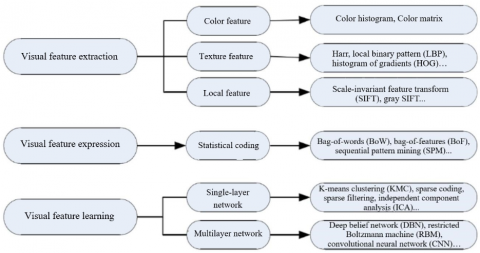Figure 1. Three methods of image feature extraction

Shallow learning techniques are often applied to image classification. Mahapatra et al.  found that some single-layer networks can learn features effectively with a few parameters. Zhao et al.  introduced a single-layer network, which is trained by unsupervised learning to learn and extract features. Single-layer networks have also been trained by unsupervised learning algorithms to learn mapping functions from k-means clustering (KMC) [5, 6], sparse coding , sparse filtering , independent component analysis (ICA) , automatic coding machine , and sparsely constrained Boltzmann machine .

In contrast to the single-layer network, deep learning requires a multilayer network structure [12,13]. The most popular deep learning frameworks are based on restricted Boltzmann machine (RBM0, deep belief network (DBN) and convolutional neural network (CNN). Singh et al.  constructed a deep learning network to process and analyze basalt images, and successfully recognized the texture features of minerals in basalt. Zhang et al.  proposed a method to segment and quantify the computed tomography (CT) scan images of rocks based on deep learning. Li et al.  trained micro-images of sandstone through deep learning, and obtained an accurate classification model for these micro-images.

In this paper, an automatic recognition framework for rock images is established based on the deep learning technique CNN and the discrete cosine transform (DCT). This framework gives full play to the good recognition ability of deep learning, while solving two main defects of the CNN in rock image classification: slow training and poor classification effect.

2. Methodology

2.1 CNN

Dedicated to processing 2D images, the CNN is a multi-layer neural network. There are three main types of layers in the network, including convolutional layer, sampling layer, and output layer. These layers are arranged in the feedforward structure: each convolutional layer is followed in turn by a sampling layer, a fully connected layer and an output layer. The convolutional and sampling layers are two-dimensional, while the output layers are one-dimensional. Each 2D layer consists of multiple planes, each of which has a 2D array of neurons. The structure of a typical CNN is shown in Figure 2, where C1 and C3 are convolutional layers and S2 and S4 are sampling layers.

In a convolutional layer (feature extraction layer), each plane is connected to one or more feature maps of the previous layer. The connection(s) is/are associated with a kernel or mask, i.e. a 2D matrix of adjustable entries called weights. Each plane calculates the convolution between its 2D input and its kernel for the first time. The convolution outputs are summed up and then added to the adjustable scalar. Finally, the activation function is applied to process the result to obtain the plane output, which is a 2D matrix called feature mapping.

Each sampling layer (feature mapping layer) has the same number of planes as the previous convolutional layer. The sampling plane divides its 2D input into non-overlapping blocks. For each block, the total value of four pixels is calculated, multiplied by an adjustable weight, and then added with a bias. Finally, the activation function is called to generate the result: an output of 2×2 blocks. Obviously, each sampling plane halves its input size along each dimension. The weights of neurons are the same in the plane of the feature mapping layer, and the feature map in the sampling layer is connected to one or more planes in the next convolutional layer.

An output layer is usually set up based on a sigmoid function or radial basis function (RBF). Here, the former is selected to build up the output layer. The outputs of this type of layers are considered the output of the entire CNN. In visual pattern classification, these outputs indicate the class of the input image.

The convolution and sampling process of the CNN is explained in Figure 3. It can be seen that the convolution process is to input the filter $f_{x}$, add a bias $b_{x}$ to the filtered result, and compute the convolution result $c_{x}$ by sigmoid function. The sampling process is to combine the adjacent four pixel values into a single value based on $b_{x}$, and apply weight $w_{x+1}$ and bias $b_{x+1}$ to the value. The sampling result $S_{x+1}$ is obtained by sigmoid function.

## 2.png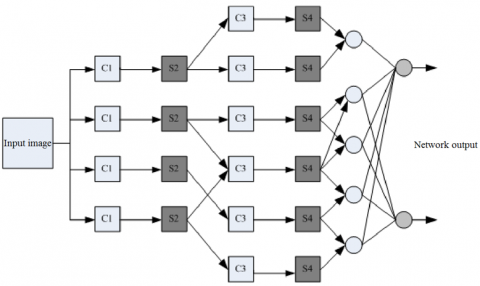Figure 2. Structure of a typical CNN

## 3.png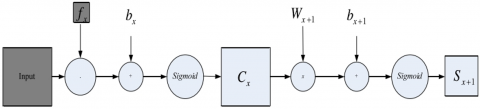Figure 3. Convolution and sampling of the CNN

## 4.pngFigure 4. Activation functions with different slopes

## 5.pngFigure 5. The operation of the convolutional layer

The sigmoid function, a common activation function of artificial neural networks (ANNs), can be expressed as:

$f(x)=\frac{1}{1+e^{-a x}}, 0<f(x)<0$  (1)

where, $\alpha$ is the slope of the function. Different activation functions have different slopes.

Figure 4 shows three activation functions with different slopes: the threshold function on the left, the sigmoid function in the middle and the hyperbolic tangent function on the right. The sigmoid function is adopted for this research.

The operation of the convolutional layer can be summed as follows: First, the feature map from the previous layer is convoluted by the kernel, and the result is processed by the sigmoid function, creating the final feature map. The convolution formula can be expressed as:

$x_{j}^{n}=f\left(\sum_{i \in j} \quad x_{i}^{n-1} * k_{i j}^{n}+b_{n}\right)$ (2)

where, $n$ is the number of network layers; k is kernel; bn  is the bias of each layer.

As shown in Figure 5, the operation of the convolutional layer is essentially to obtain neuron $x^{nj}$ of this layer using the kernel near neuron $x^{n-1}$ of the former layer, i.e. derive the feature map of this layer from that of the upper layer.

As shown in Figure 6, the operation of the sampling layer is to merge the adjacent pixels. The merging of neuron x can be described as:

$x_{j}^{n}=f\left(\frac{1}{m} \sum_{i \in j} \quad x_{i}^{n-1}+b_{n}\right)$ (3)

where, m is the size of the sampling window.

2.2 DCT

The DCT was introduced to shorten the training time and enhance the accuracy of the CNN in classification of rock images. The DCT, the real part of Fourier transform, approximates an image with a set of cosine functions (basis functions), which differ in frequency and amplitude . Therefore, the DCT can be regarded as a simplified Fourier transform.

For time series $f(x)$, where $x=0,1, \ldots, N-1$, the 1D DCT can be defined as:

$F(u)=\alpha_{0} c(u) \sum_{x=0}^{N-1} f(x) \cos \frac{(2 x+1) u \pi}{2 N}$ (4)

where, $u=0,1, \ldots N-1 ; \alpha_{0}=\frac{2}{\sqrt{N}} ; c(u)=\left\{\begin{array}{ll}{\frac{1}{\sqrt{2}}} & {u=0} \\ {1} & {u \neq 0}\end{array}\right.$.

The DCT is an orthogonal transform, capable of transforming an image from spatial domain to frequency domain. It can simplify the convolution operation, because the image is difficult to process in the spatial domain. Through the transform, the computing load is reduced and the processing speed is improved.

Through the DCT, a 2D frequency coefficient matrix can be obtained with the same size as the input image. In the matrix, the input image is represented as the linear weighted sum of a series of basis functions, which correspond to the components of the input data with different frequencies.

For an $8 \times 8$ 2D frequency coefficient matrix (Figure 6), there are 64 basis functions, which continuously increase in frequency in both horizontal and vertical directions. Therefore, after DCT transform, the lower right corner of the image is the high-frequency part, while the upper left corner is the low-frequency part.

The DCT was applied to transform an original rock image (Figure 7(a)). The image of 2D frequency coefficient matrix thus obtained is shown in Figure 7(b)). As mentioned before, the upper left corner and lower right corner of Figure 7(b) are the low-frequency part and high-frequency part, respectively. The DCT transforms the image from the time domain to the frequency domain. Although the energy remains the same, the energy distribution was changed through the transform. As shown in Figure 7(b), most of the energy gathered in the low-frequency part after the transform. After normalization, the high-frequency part contains lots of zero coefficients, revealing the good energy aggregation effect of the DCT.

## 6.pngFigure 6. An $8 \times 8$ 2D frequency coefficient matrix

## 7.pngFigure 7. DCT of the original rock image

3. Rock Image Classification Framework Based on CNN and DCT

To reduce the classification error of rock images, it is necessary for the classification framework to learn high-level features and reduce the information redundancy in the rock images.

There are lots of redundant spatial information in rock images. The pixels of a rock image are correlated to different degrees in horizontal or vertical direction. Figure 8 is the histogram of neighborhood pixel difference in the horizontal direction of Figure 7(a), where the x-axis is the difference of neighborhood pixels in the horizontal direction, and the y-axis is the number of pixels with the same neighborhood pixel difference. Obviously, 72.6% of the pixels in the rock image have a neighborhood pixel difference smaller than 20. This means the rock image has strong correlations between pixels in the horizontal direction.

According to the description of the DCT, the rock image can be transformed into the frequency domain through the DCT. Then, most information will gather in a few low-frequency coefficients in the upper left corner of the 2D frequency coefficient matrix. In fact, a few low-frequency coefficients are enough. To reduce the information redundancy of the rock image, only a few coefficients representing the image were extracted in the frequency domain, and taken as the inputs to the feature extraction module of the classification framework. As shown in Figure 9, a 1D vector was obtained by traversing the 2D frequency coefficient matrix in a zig-zag manner.

Compared with the original image, the low-frequency coefficients of the 1D vector helps the CNN to learn better high-level features in the frequency domain, laying a good basis for accurate classification. In this way, the redundancy of image information is reduced, and the DCT-CNN classification framework for rock images becomes more efficient.

## 8.pngFigure 8. Histogram of neighborhood pixel difference in horizontal direction of a rock image

## 9.png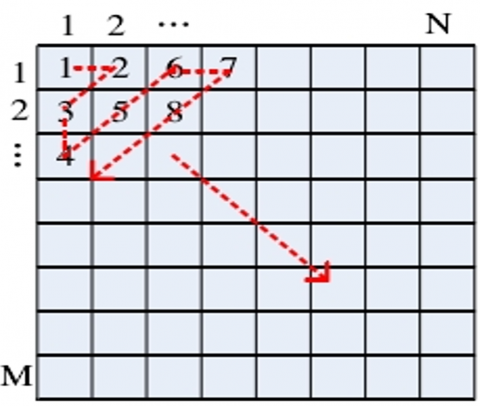Figure 9. The zig-zag scan of the 2D frequency coefficient matrix

## 10.png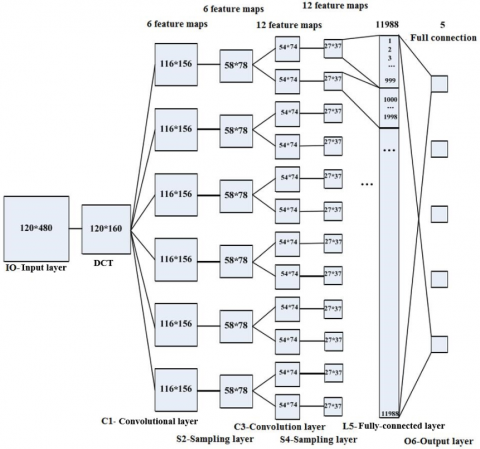Figure 10. The structure of DCT-CNN classification framework for rock images

Figure 10 presents the structure of the DCT-CNN classification framework for rock images. The framework can be established and trained in the following steps.

Step 1. Load a 120×480 training image into the input layer, and remove the hue and saturation information, leaving the image brightness only, that is, convert the RGB image into a grayscale image. Then, convert the grayscale image into a binary image, and decompose it into twenty 6×8 blocks. Perform DCT on each block and retain only the few frequency coefficients in the upper left corner in the 2D frequency coefficient matrix. The complete image after the DCT of each block is $120 \times 160$ in size.

Step 2. Forward the frequency coefficients to the first convolutional layer C1, which consists of six 5×5 neurons, a step size of 5, and a random kernel $\in[-1,1]$. The original image is convoluted from left to right and from top to bottom, a bias b (set to 0) is added to the convolution result, and six 116×156 feature maps are generated by sigmoid function. Each feature map contains the feature information of the original image.

Step 3. The nonoverlapping 2×2 sub-blocks in layer C1 are aggregated by a 2×2 filter, multiplied by a random weight w $\in[-1,1]$, added a bias b (set to 0), and six feature maps of sampling layer S2 are generated by sigmoid function. Each map is half the size of that of layer C1.

Step 4. Forward the feature maps to convolutional layer C3, which consists of six 5×5 neurons, a step size of 5, and a random kernel $\in[-1,1]$. Each feature map is convoluted from left to right and from top to bottom, a bias b (set to 0) is added to the convolution result, and twelve 2 54×74 feature maps are generated by sigmoid function.

Step 5. The nonoverlapping 2×2 sub-blocks in layer C3 are aggregated by a 2×2 filter, multiplied by a random weight w $\in[-1,1]$, added a bias b (set to 0), and twelve 27×37 feature maps of sampling layer S4 are generated by sigmoid function.

Step 6. Arrange the pixels of each of the 12 features of layer S4 from left to right and from top to bottom, and arrange 12-column pixels of the 12 maps in the order of the map. In this way, generate the fully-connected layer L5 containing 11,988 neurons. Each neuron corresponds to a pixel.

Step 7. Fully connect the 11,988 neurons of layer L5 with the five neurons of the output layer O6. The final outputs are 00001, 00010, 00100, 01000 and 10000. The, assign the corresponding rock images to categories 1~5, respectively.

The first step is equivalent to preprocessing the rock images by the DCT. Thus, the input layer and the DCT are the preprocessing module of the framework. Steps 2~5 are about feature extraction. Thus, layers C1~S4 constitute the feature extraction module. Steps 6 and 7 mainly classify the rock images, indicating that layers L5~O6 form the classification module. Through the seven steps, the working signals is propagated forward, completing the initialization and pretraining of the framework.

4. Experiment and Results Analysis

According to the study on the effect of DCT frequency coefficients on the quality of JPEG image, the fewer frequency coefficients being retained, the higher the compression ratio of the original image, yet the worse the quality of the reconstructed image. To ensure the reconstruction accuracy and control information redundancy, the number of frequency coefficients being retained should neither be too large or too small. Since the original image is split into multiple 6×8  blocks, the DCT needs to be performed on each block. In the 2D frequency coefficient matrix of each block, only the 15 frequency coefficients in the upper left corner were nonzero. Thus, only these coefficients are truly needed.

## 11.pngFigure 11. 2D frequency coefficient matrices of 6 ´ 8 blocks

In our experiment, only 10 or 15 frequency coefficients are retained from the upper-left corner of the 2D frequency coefficient matrix of each 6×8 block (Figures 10 and 11), and inputted to the DCT-CNN framework for training and testing.

Table 1 shows the test results of our framework with 10 frequency coefficients. It can be seen that our framework reduced the average error of CNN rock image classification framework by 4%.

Table 1. The test results of our framework with 10 frequency coefficients

 Image preprocessing Error of test 1 Error of test 2 Error of test 3 No preprocessing (original image) 5.03% 4.92% 5.97% Brightening 5.25% 5.19% 5.15% Darkening 5.85% 5.05% 5.20% Translation 5.35% 5.10% 5.16% Revolving 5.15% 5.18% 5.22% Mirror transform 5.20% 5.16% 5.20% Adding 0.01 intensity Gaussian noise 10.30% 10.20% 10.00% Adding 0.02 intensity Gaussian noise 15.15% 15.06% 15.17% Adding 0.01 intensity salt-and-pepper noise 11.73% 11.78% 11.60% Adding 0.02 intensity salt-and-pepper noise 17.10% 16.85% 17.95%

Table 2 provides the test results of our framework with 15 frequency coefficients. It can be seen that our framework reduced the average error of CNN rock image classification framework by 1.85% on the original image.

Table 2. The test results of our framework with 15 frequency coefficients

 Image preprocessing Error of test 1 Error of test 2 Error of test 3 No preprocessing (original image) 2.25% 2.30% 2.25% Brightening 2.65% 2.62% 2.70% Darkening 2.60% 2.55% 2.55% Translation 2.45% 2.50% 2.45% Revolving 2.55% 2.65% 2.62% Mirror transform 2.70% 2.65% 2.70% Adding 0.01 intensity Gaussian noise 7.35% 7.30% 7.40% Adding 0.02 intensity Gaussian noise 11.65% 11.60% 11.75% Adding 0.01 intensity salt-and-pepper noise 15.20% 15.28% 15.30% Adding 0.02 intensity salt-and-pepper noise 12.20% 12.25% 12.35%

As shown in Table 2, the error after brightness adjustment or geometric transform of the test image was almost equal to that for the original image.

Whichever the number of frequency coefficients, our framework consumed a similar length of training time, about 5/11 of the time consumed by the CNN rock image classification framework. Thus, our framework can greatly shorten the training time.

However, the average error of our framework changed marked after the Gaussian noise or salt-and-pepper noise was added to the original image. The error change is positively correlated with the intensity of the additive noise. This is because the image has a poor quality if the noise is intense, and the extracted features are quite ineffective. However, the error change is much smaller than that of CNN rock image classification framework, under the same conditions.

5. Conclusions

There are two defects with the CNN rock image classification framework: slow training and poor classification accuracy. The former arises from the information redundancy of the original image, and the latter comes from the lack of differentiation of rock features extracted from the spatial domain. To overcome the defects, this paper establishes a DCT-CNN classification framework for rock image, which introduces 10 to 15 frequency coefficients after the DCT to the CNN framework. Experimental results show that our framework always consumed a shorter training time and output a more accurate classification result than the CNN rock image classification framework. This means the DCT can greatly reduce the redundancy of image information.

References

 Zhang, M., Li, W., Du, Q. (2018). Diverse region-based CNN for hyperspectral image classification. IEEE Transactions on Image Processing, 27(6): 2623–2634. https://doi.org/10.1109/TIP.2018.2809606

 Foody, G.M., Mathur, A. (2004). A relative evaluation of multiclass image classification by support vector machines. IEEE Transactions on Geoscience and Remote Sensing, 42(6): 1335-1343. https://doi.org/10.1109/TGRS.2004.827257

 Mahapatra, R., Majhi, B., Rout, M. (2012). Reduced feature based efficient cancer classification using single layer neural network. Procedia Technology, 6: 180-187. https://doi.org/10.1016/j.protcy.2012.10.022

 Zhao, W., Du, S. (2016). Spectral–spatial feature extraction for hyperspectral image classification: A dimension reduction and deep learning approach. IEEE Transactions on Geoscience and Remote Sensing, 54(8): 4544-4554. https://doi.org/10.1109/TGRS.2016.2543748

 Nagi Reddy, V., Subba Rao, P. (2018). Comparative analysis of breast cancer detection using K-means and FCM & EM segmentation techniques. Ingénierie des Systèmes d’Information, 23(6): 173-187. https://doi.org/10.3166/ISI.23.6.173-187

 Chen, S., Yang, X., Tian, Y. (2015). Discriminative hierarchical k-means tree for large-scale image classification. IEEE Transactions on Neural Networks and Learning Systems, 26(9): 2200-2205. https://doi.org/10.1109/TNNLS.2014.2366476

 Zheng, M., Bu, J., Chen, C., Wang, C., Zhang, L., Qiu, G., Cai, D. (2011). Graph regularized sparse coding for image representation. IEEE Transactions on Image Processing, 20(5): 1327-1336. https://doi.org/10.1109/TIP.2010.2090535

 Goswami, G., Mittal, P., Majumdar, A., Vatsa, M., Singh, R. (2016). Group sparse representation based classification for multi-feature multimodal biometrics. Information Fusion, 32: 3-12. https://doi.org/10.1016/j.inffus.2015.06.007

 Haritopoulos, M., Yin, H., Allinson, N. M. (2002). Image denoising using self-organizing map-based nonlinear independent component analysis. Neural Networks, 15(8-9): 1085-1098. https://doi.org/10.1016/S0893-6080(02)00081-3

 Marinkovic, V., Popovic, M., Djukic, M. (2018). An automatic instruction-level parallelization of machine code. Advances in Electrical and Computer Engineering, 18(1):  27-36. https://doi.org/10.4316/AECE.2018.01004

 Karasulu, B. (2017). An optimized image segmentation approach based on Boltzmann machine. Applied Artificial Intelligence, 31(9-10): 792-807. https://doi.org/10.1080/08839514.2018.1444849

 Schmidhuber, J. (2015). Deep learning in neural networks: An overview. Neural Networks, 61: 85-117. https://doi.org/10.1016/j.neunet.2014.09.003

 Bianco, S., Buzzelli, M., Mazzini, D., Schettini, R. (2017). Deep learning for logo recognition. Neurocomputing, 245: 23-30. https://doi.org/10.1016/j.neucom.2017.03.051

 Singh, N., Singh, T. N., Tiwary, A., Sarkar, K. M. (2009). Textural identification of basaltic rock mass using image processing and neural network. Computational Geosciences, 14(2): 301-310. https://doi.org/10.1007/s10596-009-9154-x

 Zhang, J.F., Zhang, X.J., Yang, G.S. (2016). A method of rock CT image segmentation and quantification based on clustering algorithm. Journal of Xian University of Science & Technology. 36(2): 171-175. https://doi.org/10.13800/j.cnki.xakjdxxb.2016.0204

 Li, N., Hao, H., Gu, Q., Wang, D., Hu, X. (2017). A transfer learning method for automatic identification of sandstone microscopic images. Computers & Geosciences, 103: 111-121. https://doi.org/10.1016/j.cageo.2017.03.007

 Tavakoli, A., Mousavi, P., Zarmehi, F. (2018). Modified algorithms for image inpainting in Fourier transform domain. Computational and Applied Mathematics, 37(4): 5239-5252. https://doi.org/10.1007/s40314-018-0632-4